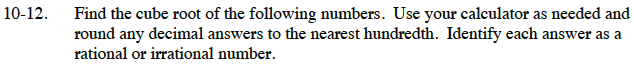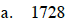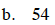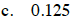Home > CC3 > Chapter Ch10 > Lesson 10.1.1 > Problem10-12

10-12.
1. Find the cube root of the following numbers. Use your calculator as needed and round any decimal answers to the nearest hundredth. Identify each answer as a rational or irrational number. Homework Help ✎

1. 1728

2. 54

3. 0.125If this was the volume of a cube, how long would its side be?Consider the same situation from part (a).

Irrational numbers are cube roots of non-perfect cubes.

3.78, irrationalSee parts (a) and (b).